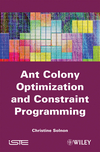# Ant Colony Optimization and Constraint Programming

 Wiley-ISTE, ISBN: 978-1-84821-130-8, 232 pages Associated programs: AntSolver   AntClique    AntCar• Forewords of Pascal Van Hentenryck
• Chapter 1. Introduction
• Chapter 2. Computational Complexity
• 2.1. Complexity of an algorithm
• 2.2. Complexity of a problem
• 2.3. Where the most difficult instances can be found
• 2.4. Solving NP-hard problems in practice

PART I. CONSTRAINT PROGRAMMING

• Chapter 3. Constraint Satisfaction Problems
• 3.1. What is a constraint?
• 3.2. What is a constraint satisfaction problem?
• 3.3. Optimization problems related to CSPs
• 3.4. Examples
• 3.5. Discussion
• Chapter 4. Exact Approaches
• 4.1. Construction of a search tree
• 4.2. Constraint propagation
• 4.3. Ordering heuristics
• 4.4. From satisfaction to optimization problems
• 4.5. Discussion
• Chapter 5. Perturbative Heuristic Approaches
• 5.1. Genetic algorithms
• 5.2. Local search
• 5.3. Particle swarm optimization
• 5.4. Discussion
• Chapter 6. Constructive Heuristic Approaches
• 6.1. Greedy randomized approaches
• 6.2. Estimation of distribution algorithms
• 6.3. Ant colony optimization
• 6.4. Discussion
• Chapter 7. Constraint Programming Languages
• 7.1. Constraint logic programming
• 7.2. Constraint programming libraries
• 7.3. Constraint-based local search
• 7.4. Discussion

PART II. ANT COLONY OPTIMIZATION

• Chapter 8. From Swarm Intelligence to Ant Colony Optimization
• 8.1. Complex systems and swarm intelligence
• 8.2. Searching for shortest paths by ant colonies
• 8.3. Ant system and the traveling salesman problem
• 8.4. Generic ACO framework
• Chapter 9. Intensification versus Diversification
• 9.1. ACO mechanisms for intensifying the search
• 9.2. ACO mechanisms for diversifying the search
• 9.3. Balancing intensification and diversification
• 9.4. Measures of diversification/intensification
• Chapter 10. Beyond Static Combinatorial Problems
• 10.1. Multi-objective problems
• 10.2. Dynamic optimization problems
• 10.3. Optimization problems over continuous domains
• Chapter 11. Implementation Issues
• 11.1. Data structures
• 11.2. Selection of a component with respect to probabilities
• 11.3. Implementation of a local search procedure
• 11.4. Computation of diversification/intensification measures

PART III. CP WITH ACO

• Chapter 12. Sequencing Cars with ACO
• 12.1. A first pheromone structure for identifying good car sequences
• 12.2. A second pheromone structure for identifying critical cars
• 12.3. Combining the two pheromone structures
• 12.4. Experimental evaluation
• 12.5. Discussion
• Chapter 13. Subset Selection with ACO
• 13.1. Subset selection problems
• 13.2. Description of Ant-SSP
• 13.3. Instantiations of Ant-SSP with respect to two pheromone strategies
• 13.4. Instantiation of Ant-SSP to solve CSPs
• 13.5. Experimental results
• 13.6. Discussion
• Chapter 14. Integration of ACO in a CP Language
• 14.1. Framework for integrating ACO within a CP library
• 14.2. Illustration of ACO-CP on the car sequencing problem
• 14.3. Discussion
• Chapter 15. Conclusion
• Bibliography